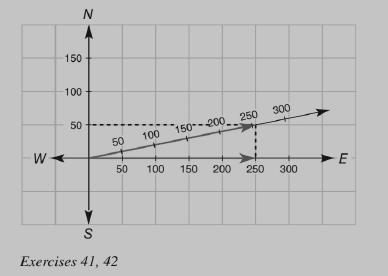Chapter 4.1, Problem 41EElementary Geometry For College St...

7th Edition
Alexander + 2 others
ISBN: 9781337614085

Solutions

Chapter
SectionElementary Geometry For College St...

7th Edition
Alexander + 2 others
ISBN: 9781337614085
Textbook Problem

The following problem is based on the Parallelogram Law. In the scaled drawing, each unit corresponds to 50 mph. A small airplane travels due east at 250 mph. The wind is blowing at 50 mph in the direction due north. Using the indicated diagonal and use it to determine the speed of the airplane in miles per hour.To determine

The speed of the airplane in miles per hour using the indicated diagonal.

Explanation

Given:

In the scaled drawing given below, each unit corresponds to 50 mph. A small airplane travels due east at 250 mph. The wind is blowing at 50 mph in the direction due north.

Parallelogram Law:

Enables to determine the speed and direction of an airplane when the velocity of the airplane and that of the wind are considered together...

Still sussing out bartleby?

Check out a sample textbook solution.

See a sample solution

The Solution to Your Study Problems

Bartleby provides explanations to thousands of textbook problems written by our experts, many with advanced degrees!

Get Started

Find all possible real solutions of each equation in Exercises 3144. y3+64=0

Finite Mathematics and Applied Calculus (MindTap Course List)

Fill in each blank: 6lb9oz=oz

Elementary Technical Mathematics

What is wrong with the equation? 0sec2xdx=tanx]0=0

Single Variable Calculus: Early Transcendentals

The slope of the tangent line to r = cos θ at is:

Study Guide for Stewart's Multivariable Calculus, 8th

The average value of f(x) = 3x2 + 1 on the interval [2, 4] is: a) 29 b) 66 c) 58 d) 36

Study Guide for Stewart's Single Variable Calculus: Early Transcendentals, 8th